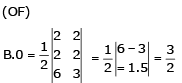Courses

# Test: Chemical Bonding and Molecular Structure- 2

## 30 Questions MCQ Test Chemistry for JEE | Test: Chemical Bonding and Molecular Structure- 2

Description
This mock test of Test: Chemical Bonding and Molecular Structure- 2 for Class 11 helps you for every Class 11 entrance exam. This contains 30 Multiple Choice Questions for Class 11 Test: Chemical Bonding and Molecular Structure- 2 (mcq) to study with solutions a complete question bank. The solved questions answers in this Test: Chemical Bonding and Molecular Structure- 2 quiz give you a good mix of easy questions and tough questions. Class 11 students definitely take this Test: Chemical Bonding and Molecular Structure- 2 exercise for a better result in the exam. You can find other Test: Chemical Bonding and Molecular Structure- 2 extra questions, long questions & short questions for Class 11 on EduRev as well by searching above.
QUESTION: 1

### There is no S-S bond in

Solution: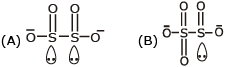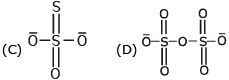QUESTION: 2

### Which of the following molecules are expected to exhibit intermolecular H-bonding I. Acetic acid II. o-nitrophenol III. m-nitrophenol IV. o-boric acid

Solution: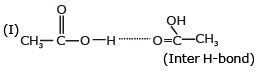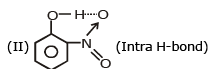QUESTION: 3

### A : tetracyanomethane                           B : Carbondioxide C : Benzene                                           D : 1, 3-buta-di-ene Ratio of σ and π bond is in order:

Solution:

(A) Tetra cyanomethane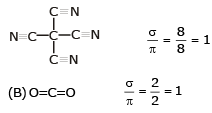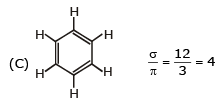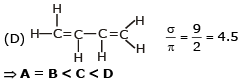QUESTION: 4

Which of the following models best describes the bonding within a layer of the graphite structure ?

Solution: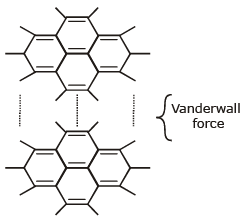QUESTION: 5

In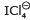, the shape is square planer. The number of bond pair-lone pair repulsion at 90° are :

Solution: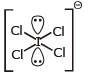⇒ No of bond pair-lone pair repulsion = 8

QUESTION: 6

In which of the following molecules / ions all the bonds are not of equal length.

Solution: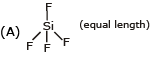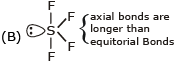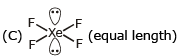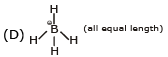QUESTION: 7

The critical temperature of water is higher than that of O2 because the H2O molecules has :

Solution:

⇒ H2O is polar molecule
⇒ Hence  H2O has higher critical temp

QUESTION: 8

Compound with maximum ionic character is formed from :

Solution:

for maximum ionic character,
* IE of cation must be less
* EA of anion must be larger
⇒ CS and  F
⇒ (B)

QUESTION: 9

Mg2C3 reacts with water forming propyne, C3 4- has

Solution: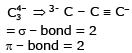QUESTION: 10

Which of the following has a geometry different from the other three species (having the same geometry)?

Solution: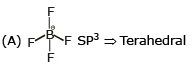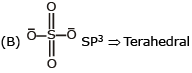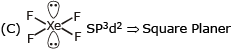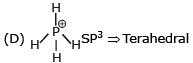QUESTION: 11

Which of the following molecule does not have open book structure.

Solution: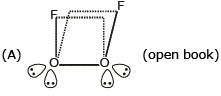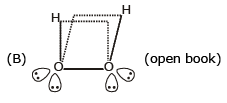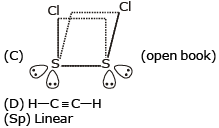QUESTION: 12

The hybridisation and geometry of BrF3 molecules are :

Solution: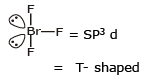QUESTION: 13

Pick out the incorrect statement ?

Solution:

(A) N2 B.O = 3 B.O α stability
N2+ B.O = 2.5
Correct Statement
(B) O2 ⇒ B.O = 2
O2 ⇒ B.O = 2.5 ⇒ Correct Statement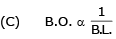(D) NO+, B.O. = 3
NO, B.O = 2.5
= Correct statement

QUESTION: 14

Ethanol has a higher boiling point than dimethyl ether though they have the same molecular weight. This is due to :

Solution: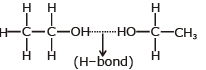⇒ due to intermolecular H-bond, it has higher b.p. than CH3OCH3

QUESTION: 15

A simplified application of MO theory to the hypothetical ‘molecule’ OF would give its bond order as

Solution: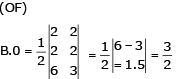QUESTION: 16

Which of the following contains electrovalent and covalent bonds ?

Solution: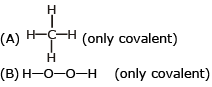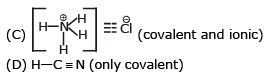QUESTION: 17

Which of the following species is paramagnetic ?

Solution: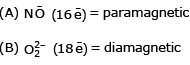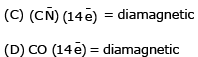QUESTION: 18

Bond order of Be2 is

Solution: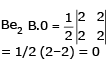QUESTION: 19

The volatility of HF is low because of :

Solution:

due to H–bond,
HF has Low volatility

QUESTION: 20

Which of the following sets of characteristics leads to the increase in solubility of ionic substances ?

Solution:

High dipole moment and strong attraction by ions means that they can form intermolecular Hydrogen bond with water molecules. High solvation energy means that when the compound is dropped into water, then when the compound forms H-bond with water, then a high amount of energy is released. This is favourable to solubility as more heat is released, more the compound becomes soluble.

QUESTION: 21

Dipole-induced dipole interactions are present in which of the following pairs?

Solution:

Dipole-induced dipole interaction is present in the pair in which the first species is polar and the other is non-polar.
H2O and alcohol both are non-polar so there exist dipole-dipole interactions in between them.
Cl2 and CCl4 both are non-polar so there exists induced dipole -induced dipole interactions in between them. Similarly is true for SiCl4 and He atoms pair.

HCl is a polar molecule, whereas He atoms are non-polar, so in between them dipole-induced dipole interactions exist.

QUESTION: 22

Which one of the following conversions involve change in both hybridization and shape?

Solution:

Hybridisation -

The process of mixing of atomic orbitals belonging to the same atoms of slightly different energies so that a redistribution of energy takes place between them  resulting in the formation of new set of orbital of equivalent energies and shape is called hybridisation.- wherein The new orbitals thus formed are called hybrid orbitals.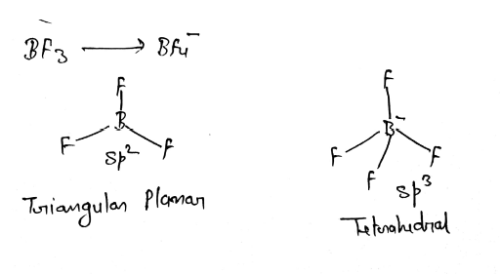QUESTION: 23

The molecule is not having 3c-2e bond.

Solution: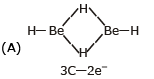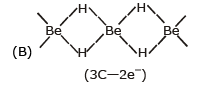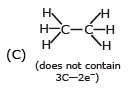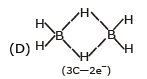QUESTION: 24

Among KO2, AlO-2 ,BaO2 and NO+2 unpaired electron is present in :

Solution: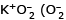contain one unpaired electron so paramagnetic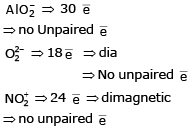QUESTION: 25

Which has maximum dipole moment ?

Solution: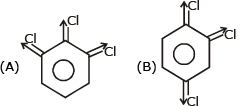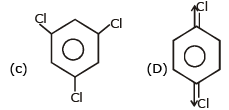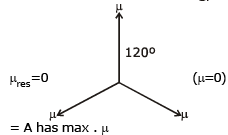QUESTION: 26

Ethanol has a higher boiling point than dimethyl ether though they have the same molecular weight. This is due to :

Solution: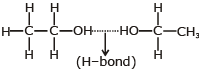⇒ due to intermolecular H-bond, it has higher b.p. than CH3OCH3

QUESTION: 27

Arrange the following in order of decreasing boiling point :

(I) n-Butane (II) n-Butanol (III) n-Butyl chloride (IV) Isobutane

Solution:

(I) H3C—CH2—CH2—CH3 n-butane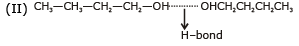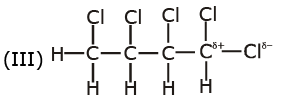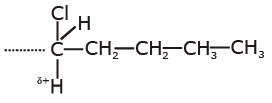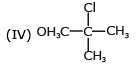II > III >I > IV
As H-bond>d Interaction
> st. chain > Branch chain

QUESTION: 28

Which of the following compounds would have significant intermolecular hydrogen bonding ?

HF, CH3OH, N2O4, CH4

Solution: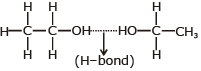⇒ due to intermolecular H-bond, it has higher b.p. than CH3OCH3

QUESTION: 29

For H2O2, H2S, H2O and HF , the correct order of decreasing extent of hydrogen bonding is :

Solution: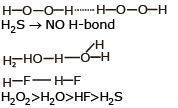QUESTION: 30

A simplified application of MO theory to the hypothetical `molecule' OF would give its bond order as

Solution: